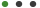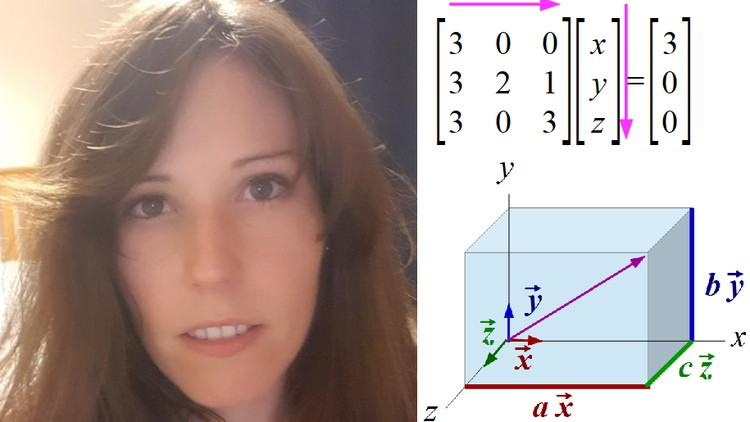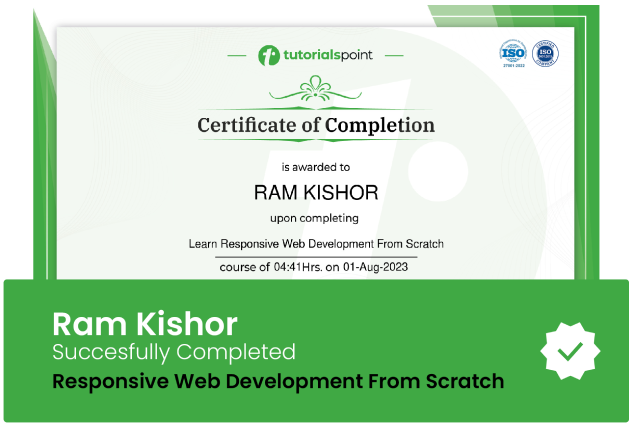### Algebra (vectors and matrices) in MathematicsAlison C

# Algebra (vectors and matrices) in Mathematics

Transpose, Determinant, Adjoint, Eigenvalues, Eigenvectors, DiagonalizeUpdated on Nov, 2023Language - EnglishAlison CMathematics,Algebra,Vector & calculas,Teaching & Academics

Lectures -16

Resources -15

Duration -1.5 hours30-days Money-Back Guarantee

Training 5 or more people ?

## Course Description

Hi!

My name is Alison

I'm an analytical person with a science background, that has a passion for complex problem solving. I have a PhD in Science and I currently work as a Software Developer.

I'm here to teach you Maths, Chemistry, Physics, and Programming.

I present my courses in a clear, logical, and structured way. For every topic, I also include an example or exercise to make sure that it is clear and easy to understand.

Please send me your feedback and let me know if you have any comments, questions, or suggestions. I may be able to prepare a course that interests you.

In this specific course, we will learn various concepts about algebra in mathematics

1) First, we will introduce what is a vector, its properties, and how we can represent it in both one and three dimensions. We will also talk about unit vectors.

2) Afterwards, we will explain what is a linear combination of vectors and how we can solve these equations if the vectors are independent or dependent.

3) we will introduce what is a matrix with an example to understand this

4) We will learn how to calculate the transpose, determinant and adjoint of a matrix with examples

5) Then, we will explain what are the eigenvalues and eigenvectors of a matrix as well as how we can calculate them

6) Finally, I will show you how we can obtain the diagonal of a matrix using the eigenvalues and eigenvectors

I hope you enjoy this course, find it easy and learn a lot with me

Good luck and see you soon

Alison

### Goals

What will you learn in this course:

• What is a vector and its properties both in one and three dimensions

• What are unit vectors

• What are linear combinations of vectors (both independent and dependent)

• What are matrices

• How to calculate the transpose, determinant and adjoint of a matrix

• What are the eigenvectors and eigenvalues of a matrix and how we can calculate them

• How to obtain the diagonal of a matrix using the eigenvectors and eigenvalues

### Prerequisites

What are the prerequisites for this course?

• Interest to learn basic mathematics
• A paper and pen to try all the examples and exercises
• Basic and intermediate mathematics learners
• University students
• GCSE students
• People interested in learning maths## Curriculum

Check out the detailed breakdown of what’s inside the course

Introduction
1 Lectures
•Introduction 01:27 01:27
Content of this course
1 LecturesWhat is a vector, its properties and what are unit vectors
1 LecturesLinear combination of vectors (independent and dependent)
1 LecturesWhat is a matrix
2 LecturesTranspose of a matrix
1 LecturesDeterminant of a matrix
1 Lectures2 LecturesEigenvectors and Eigenvalues of a matrix
4 LecturesDiagonal of a matrix
2 Lectures## Instructor DetailsAlison C

Hi!

My name is Alison

I'm an analytical person with a science background, that has a passion for complex problem solving. I have a PhD in Science and I currently work as a Software Developer.

I'm here to teach you Maths, Chemistry, Physics and Programming.

I present my courses in a clear, logical and structured way. For every topic, I also include an example or exercise to make sure that it is clear and easy to understand.

Please send me your feedback and let me know if you have any comments, questions or suggestions. I may be able to prepare a course that interests you.

I hope you enjoy my courses, find them easy and learn a lot with me

All the best and see you soon

Alison

## Course Certificate

User your certification to make a career change or to advance in your current career. Salaries are among the highest in the world.